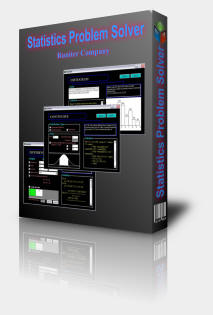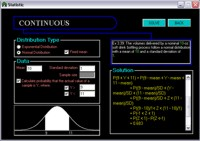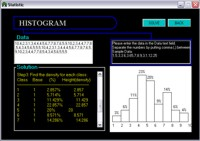Statistics Problem Solver 2.2

 Product Overview How to use Screen shots What's new

 Get itAre you taking a beginner statistics course and need help in learning statistics and solving your statistics homework? This is statistics tutorial software that can solve statistical problems and generate step-by-step solutions.

# Statistics Tutorial...

Statistics help is only a few clicks away. This amazing computer program not only solves your statistical problems for you, but it also shows you how to solve them by demonstrating the various steps and formulas that are involved in solving those problems.

# Easy to get Statistics Help...Getting statistics help to learn more about statistical distributions or to do your statistics homework is easy. Simply select one of the available statistical distributions from the main menu (i.e. continuous distribution). Then fill in the blanks with data given by the problem statement that you are trying to solve. Finally press Solve and observe the step-by-step solution that is generated for you.

# Statistics tutorial for beginners...This software is intended to be a statistics help for university and college students who are taking beginner statistics courses. Statistic Problem Solver is designed based on University of Ottawa Statistics & Probability (MAT2377) course. The solution algorithms are based on those introduced in the "Statistical Methods for Engineers" book by G. Geoffrey Vining, Duxbury, 1998, and also the lecture notes of professor D. McDonald's.

### Key Features

The current version of Statistics Problem Solver can solve and generate the following statistical analysis:

• Plotting Histograms: The histogram generator inputs numbers as data and draws a histogram based on the given data.
• Discrete Distributions: Two types of distributions can be analyzed here:
• Binomial Distribution
• Poisson Distribution
• Continuous distributions: Continuous distributions can be solved here based on one of the following distributions:
• Exponential Distribution
• Normal Distribution
• Test of Significance: Test your hypotheses using the significance test analyzer and figure out whether the mean of a give information is what you expected.
• Data Comparison: Compare two groups of statistical data in order to test your hypothesis of the differences between the groups.
 60-day Money Back Guarantee: We care about your satisfaction and for that, all our products are subject to a 60-day money back guarantee. If for any reason you were not satisfied with a purchased product, we will refund you within 24 hours of receiving your refund request. For refund, email us at support@runiter.com with your name and order id.

See our other products:

Copyright (c) 2004 - Runiter Company - support@runiter.com